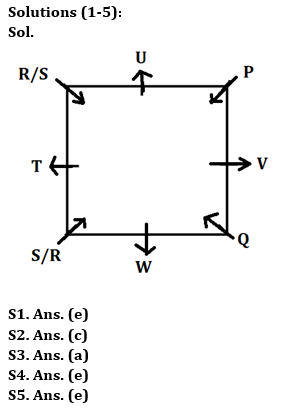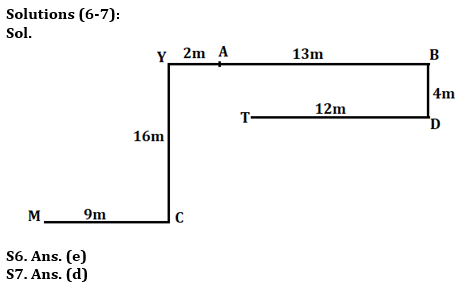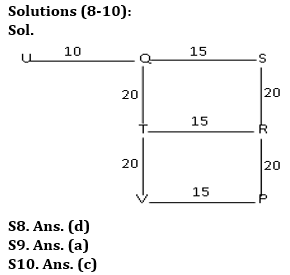Latest Banking jobs   »     »

# Reasoning Quizzes Quiz For IBPS RRB PO/Clerk Prelims 2023-27th May

Directions (1-5): Answer the questions based on the information given below.
Eight persons P, Q, R, S, T, U, V and W sit around a square table such that four of them sit at the corner of the table and facing towards the center whereas four of them sit at the middle of the side of the table and facing away the center but not necessarily in the same order.
P sits 3rd to the left of W. Neither R nor S sits adjacent to P. T sits 3rd to the right of P. Three persons sits between T and V. Q sits immediate right of V but not at the middle of the sides.

Q1. Who sits 3rd to the right of S?
(a) W
(b) P
(c) U
(d) Q
(e) Can’t be determined

Q2. Who sits 2nd to the left of V?
(a) P
(b) W
(c) U
(d) R
(e) None of these

Q3. How many persons sit between P and Q when counted from the left of Q?
(a) Five
(b) One
(c) Four
(d) Three
(e) None of the above

Q4. What is the position of R with respect to W?
(a) Immediate left
(b) Immediate right
(c) 3rd to the right
(d) 2nd to the left
(e) Either (b) or (c)

Q5. Four of the following are similar in a certain way and forms a group. Find out the one who does not belong to that group.
(a) P
(b) Q
(c) R
(d) S
(e) T

Directions (6-7): Study the given information and answer the following question.
Point A is 13m west of point B. Point D is 4m south of point B. Point C is 9m east of point M. Point T is 12m west of point D. Point Y is 16m north of point C. Point Y is 2m west of point A.

Q6. What is the direction of point C with respect of point T?
(a) North-west
(b) South
(c) North-east
(d) West
(e) None of these

Q7. In which direction is point A with respect to Point T?
(a) North
(b) North-east
(c) East
(d) North-west
(e) None of these

Directions (8-10): Study the following information carefully and answer the given questions.
Point S is 40 m towards the North of Point P. Point Q is 15 m towards the West of point S. Point U is 25 m towards the West of point S. Point T is 20 m towards the South of point Q. Point R is 15 m towards the East of point T. Point V is 15 m towards the West of point P.

Q8. Which of the following points are in a straight line?
(a) S, T, P
(b) T, V, R
(c) S, Q, V
(d) T, V, Q
(e) U, Q, R

Q9. Point P is in which direction with respect to point R?
(a) South
(b) North
(c) East
(d) West
(e) Cannot be determined

Q10. If a person walks 10 m towards East from point U and then takes a right turn, which of the following points would he reach first?
(a) V
(b) S
(c) T
(d) P
(e) R

Solutions## FAQs

### What is the selection process of IBPS RRP PO?

There are 4 sections in the IRDAI assistant manager online preliminary exam. They are Reasoning, English Language, General awareness, Quantitative aptitude.

#### Congratulations!Union Budget 2023-24: Free PDF